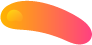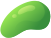##### Derive the moment generating function

A technology company has two independent servers. Denote X as the downtime in hours of server 1 and denote Y as the downtime in hours of server 2. X follows an exponential distribution with parameter β > 0 and Y follows an exponential distribution with parameter α > 0, where β and α are both finite.

Assume X and Y are independent of one another.
a. What is the probability that server 1 will be down at least as long as server 2 is down? Show

work.
b. What is E[X(X + Y )] and var(X + Y )? Show work and reasoning.

c. Derive the moment generating function for server 1’s downtime (X) and use it to get the third moment of X (that is get E(X3)). Show work.

d. Derive the moment generating function for the combined down time across server 1 and server 2, X +Y. Show work.

e. Now say we have a sequence of n many servers such that the downtime on server n, Xn, follows an exponential distribution with parameter n.
Show that Xn converges in probability to 0 as n goes to infinity